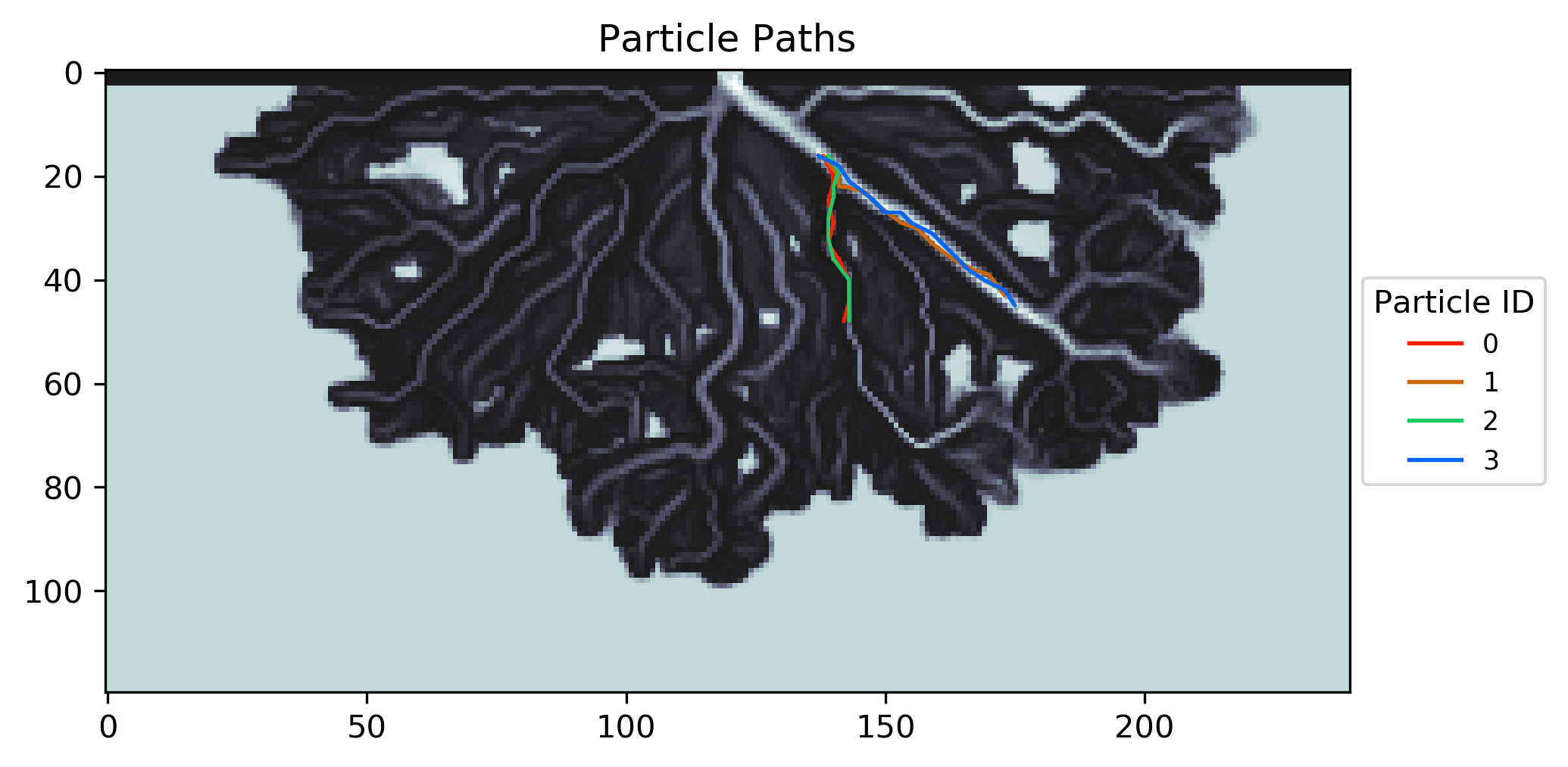# Example 7 - Drawing Particle Travel Paths (DeltaRCM)¶

In this example, we will use the particles movement simulated in Example 2 - Particles in Steady Flow (DeltaRCM), and visualize some of the particle travel paths.

Full example script available `here`.

First run Example 2 - Particles in Steady Flow (DeltaRCM), and then we can access and use the particle travel information output by that example.

```>>> import numpy as np
>>> import json
>>> import os
```>>> draw_travel_path(depth, all_walk_data, [0, 1, 2, 3],